Torsion of Shafts

# Torsion of Shafts - Notes | Study Mechanical Engineering SSC JE (Technical) - Mechanical Engineering

 1 Crore+ students have signed up on EduRev. Have you?

TORSION OF SHAFTS

TORSION OF CIR CULAR  SHAFTS
Theory of Pure Torsion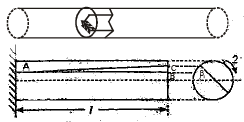Torsional Moment of Resistance: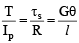ts= Shear intensity at the surface of the shaft
G = Modulus of rigidity of shaft material
l = Length of shaft
q = Angular movement due to strain in length of the shaft
T = total moment of resistance offered by the cross-section of the shaft
I|p= Polar moment of Inertia of the section of the shaft

Assumptions in the theory of pure torsion: 

• The material of the shaft is uniform throughout. 
• Twist along the shaft is uniform. 
• Shaft is of uniform circular section throughout, which may be hollow or solid. 
• Cross section of the shaft, which are plane before  twist remain plane after twist. 
• All radii which are straight  before twist remain straight after twist:

Polar modulus:

Polar modulus =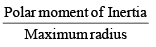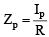The greatest twisting moment which a given shaft section can resist = Max. permissible shear stress × Polar Modulus

T = ts Zp

• For solid shaft,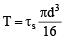for hollow shaft,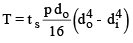Where, do= outer diameter
di = inner diameter
Torsional rigidity: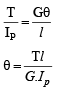Where, G = rigidity modulus
Ip = Polar moment of Inertia
The quantity GIp is called torsional rigidity. It is the torque required to produce a twist of 1 radian per unit length of the shaft.

Power Transmitted by a shaft: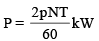= Torque × angle turned per second
Where, P = Power transmitted (kW)
N = rotation per minute (rpm)
T = mean torque (kNm)

SHAFTS IN SERIES AND SHAFTS IN PARALLEL
(a) shafts in series:
• Torque T will be same for both the shafts.
• The twists 1q and 2q will be different for both the shafts.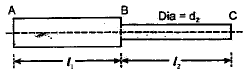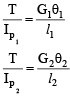Where, T = Torque
G1, G2 = Modulus of rigidity for shafts 1& 2
l1, l2 = length of shaft 1&2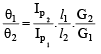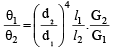if l1 = l2 G1 = G2 them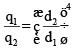Where, q1,q2 = angleof twi,st
Ip1, Ip2 = polar moments of inertia

(b) Shafts in parallel:

•  In this case applied torque T is distributed to two shafts.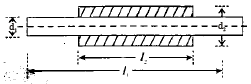• T = T1 + T2
• The angle of twist will be same for each shaft,

q1 = q2 = q

• T = T1 + T2 =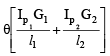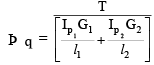COMPARISON BETWEEN SOLID AND HOLLOW SHAFTS
Let hollow shaft and solid shafts have same material and length.
D0 = external diameter of hollow shaft
Di = nD= Internal diameter of hollow shaft
D = Diameter of the solid shaft

Case (i): When the hollow and solid shafts have the same torsional strength.

• In this case polar modulus section of two shafts would be equal.
•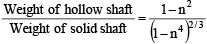• USE: %Saving in weight can be calculated for same torsional strength.

Case (ii): When the hollow and solid shafts are of equal weights.

• In this case torsional strength is compared.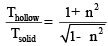• USE: ratio of strength for same weight can be calculated.

Case (iii) : When the diameter of solid shaft is equal to the external diameter of the hollow shaft.

•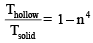SHEAR AND TORSIONAL RESILIENCE
Shear resilience:
Let t = shear stress intensity at faces of a square block

• Strain energy stored per unit volume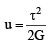= (uniform through the section)
Where G = rigidity modulus.

Torsional resilience:

• In this case shear stress due to torsion varies uniformly form zero at the axis to the maximum value ts at the surface.
• Strain energy stored, per unit volume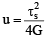• for hollow shaft,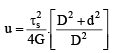Where, D = outer diameter of hollow shaft
d = internal diameter of hollow shaft

The document Torsion of Shafts - Notes | Study Mechanical Engineering SSC JE (Technical) - Mechanical Engineering is a part of the Mechanical Engineering Course Mechanical Engineering SSC JE (Technical).
All you need of Mechanical Engineering at this link: Mechanical Engineering

## Mechanical Engineering SSC JE (Technical)

6 videos|97 docs|55 tests
 Use Code STAYHOME200 and get INR 200 additional OFF

## Mechanical Engineering SSC JE (Technical)

6 videos|97 docs|55 tests

Track your progress, build streaks, highlight & save important lessons and more!

,

,

,

,

,

,

,

,

,

,

,

,

,

,

,

,

,

,

,

,

,

;